Browse by Topic
Related Topics

# New Derivatives of the Bessel Functions Have Been Discovered with the Help of the Wolfram Language!

##### Nearly two hundred years after Friedrich Bessel introduced his eponymous functions, expressions for their derivatives with respect to parameters, valid over the double complex plane, have been found.

In this blog we will show and briefly discuss some formerly unknown derivatives of special functions (primarily Bessel and related functions), and explore the history and current status of differentiation by parameters of hypergeometric and other functions. One of the main formulas found (more details below) is a closed form for the first derivative of one of the most popular special functions, the Bessel function J: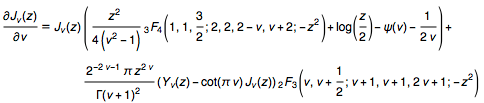Many functions of mathematical physics (i.e. functions that are used often and therefore have special names) depend on several variables. One of them is usually singled out and designated as the “argument,” while others are usually called “parameters” or sometimes “indices.” These special functions can have any number of parameters. For example (see the Wolfram Functions Site), the Bessel functions Jv(z) and Iv(z), the Neumann function Yv(z), the Macdonald function Kv(z), and the Struve functions Hv(z) and Lv(z) take only one parameter (called the index), while the Whittaker functions Mμ,v(z) and Wμ,v(z) as well as the confluent hypergeometric functions 1F1(a; b; z) and U (a, b, z) take two parameters. The Anger functions Jv(z) and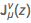as well as the Weber functions Ev(z) andcan have one or two parameters (in the cases of two parameters, they are called generalized Anger and Weber functions). The Appell and Humbert functions mostly have from three to five parameters, while more complicated special functions such as the generalized hypergeometric function pFq(a1, …, ap; b1, …, bq; z) can have any finite number of parameters.

Among other properties, differentiation of special functions plays an essential role, since derivatives characterize the behavior of functions when these variables change, and they are also important for studying the differential equations of these functions. Usually, differentiation of a special function with respect to its argument presents no essential difficulties. The largest collection of such derivatives, comprising the first, second, symbolic, and even fractional order for 200+ functions, can be found in the section “Differentiation” at the Wolfram Functions Site (for example, see this section, which includes 21 such derivatives for the Bessel function Jv(z)), or Y. A. Brychkov’s Handbook of Special Functions). The majority of these formulas are also available directly in the Wolfram Language through the use of the built-in symbols MathematicalFunctionData and EntityValue.

Derivatives with respect to parameters (as distinct from the argument), however, can generally be much more difficult to compute; that is the subject of this blog. Remarkably, the formula above, involving the generic first-order (with respect to the single parameter v) derivative of one of the most commonly occurring special functions in mathematical physics, has only just been discovered in closed form, and this perhaps surprising fact speaks to the difficulty of the general problem. So, using the Bessel J function as a characteristic example, let us take a brief walking tour through the history of special function differentiation.

#### Derivatives aren’t easy

Often, people even well acquainted with calculus tend to think that integration is difficult and differentiation is easy. The folklore is that “differentiation is mechanics, integration is art.” But the spirit of this saying is true only for elementary functions, where differentiation again produces elementary functions (or combinations thereof). For hypergeometric and other lesser-known special functions, when differentiation is carried out with respect to parameters, it can typically produce complicated functions of a more general class.

The distinction between differentiation with respect to parameters versus differentiation with respect to argument is exemplified by the Bessel J function. The derivative of Bessel J with respect to its argument z has been known for quite some time, and has this relatively simple closed form: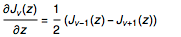However, the analytic evaluation of its derivative with respect to parameters (e.g. v in the above equation) is more complicated. Often, derivatives such as these can be written in the form of an integral or infinite series, but those objects cannot be represented in closed form through other simple or well-known functions. Historically, some special functions were introduced for the sole purpose of giving a simple notation for the derivatives of other, more basic functions. For example, the polygamma function arose in this way as a means of representing derivatives of the gamma function.

The generalized hypergeometric function pFq(a1, …, ap; b1, …, bq; z) and its derivatives play an essential role in the solution of various problems in theoretical and applied mathematics (see, for example, this article by L. U. Ancarani and G. Gasaneo concerning the applications of derivatives by parameters in quantum mechanics). The generalized hypergeometric function generates as special cases many of the most-used elementary functions (e.g. the trigonometric, hyperbolic, logarithmic, and inverse trigonometric functions) as well as many families of more specialized functions, including the Bessel, Struve, Kelvin, Anger–Weber, incomplete gamma, and integral (exponential, sine, and cosine) functions. In the case p = 0, q = 1, the generalized hypergeometric function pFq subsumes the Bessel family of functions Jv(z), Iv(z), Yv(z), and Kv(z). To be precise, Bessel J, for example, has the following hypergeometric representation: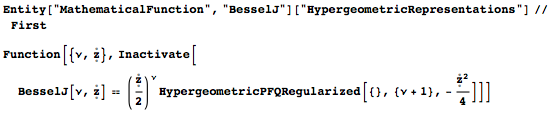Interestingly, the history of the function Jv(z) starts nearly exactly 200 years ago. In the 1816–17 proceedings of the Berlin Academy (published in 1819), in the work Analytische Auflösung der Keplerschen Aufgabe, Friedrich Wilhelm Bessel deals with the so-called Kepler equation
M=Ee sin(E), where M is the mean anomaly, E is the eccentric anomaly, and e is the eccentricity of a Keplerian orbit. The solution of this equation can be represented (in today’s notation) through Bessel functions of integer order: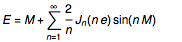In this first work, Bessel does not yet use the modern notation, but his function appears already implicitly. For example, he uses the following sum (note that Bessel uses Gauss’ notation 𝜋i for i!):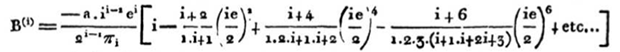In modern times, we could write this as the sum of two Bessel functions, which can be shown in the Wolfram Language: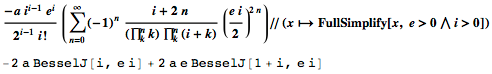Furthermore, this sum is just the first derivative of the single Bessel function -2 a e J(i, e i):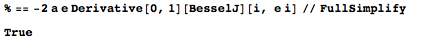In a second work from 1824, Bessel uses the nearly modern notation (with JI) to denote his function: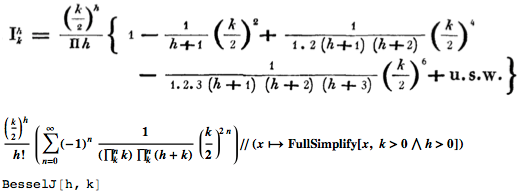He also derives fundamental relations for this function: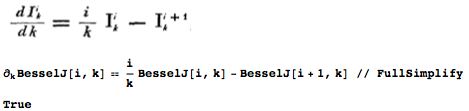Various special instances of the generic Bessel function occur already in the writings of Bernoulli, Euler, d’Alembert, and others (see this article for a detailed account). The main reference about Bessel functions today is still the classical monograph by G. N. Watson, A Treatise on the Theory of Bessel Functions, which has been republished and extended many times since 1922.

So while the derivatives of Bessel J with respect to the argument z were known since the beginning of the nineteenth century, it took until the middle of the twentieth century before special cases for derivatives with respect to the index v were found. The derivatives of some Bessel functions with respect to the parameter v at the points v ==0, 1, 2,… and v == 1/2 were obtained by J. R. Airey in 1935, and the expressions for other Bessel family functions were given by W. Magnus, F. Oberhettinger, and R. P. Soni in “Formulas and Theorems for the Special Functions of Mathematical Physics” (1966):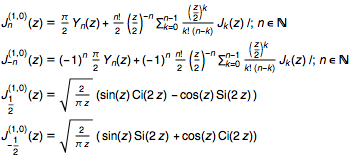Generalizations to any half-integer values of v were reported more recently at an international conference on abstract and applied analysis (Hanoi, 2002) as the following: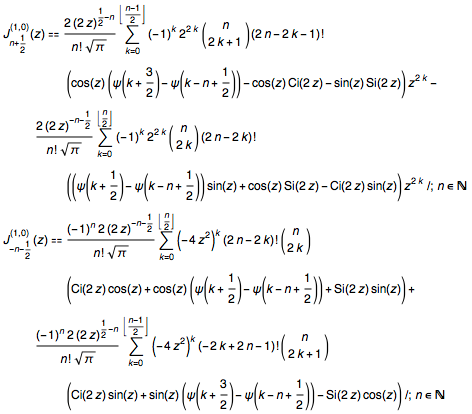These results, along with expressions for the parametric derivatives of Struve functions at integer and half-integer values, were published in 2004–2005. Various new formulas for differentiation with respect to parameters of the Anger and Weber functions, Kelvin functions, incomplete gamma functions, parabolic cylinder functions, Legendre functions, and the Gauss, generalized, and confluent hypergeometric functions can be found in the Handbook of Special Functions: Derivatives, Integrals, Series and Other Formulas. For a short survey and references, see H. Cohl.

But perhaps amazingly, given all this work, the first derivatives of the Bessel functions in closed form for arbitrary values of the parameter were obtained only in 2015 (Y. A. Brychkov, “Higher Derivatives of the Bessel Functions with Respect to the Order” (2016)). They are expressed as combinations of products of Bessel functions and generalized hypergeometric functions; for example: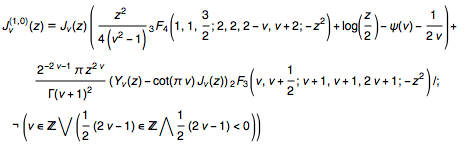The plots below give some impressions about the behavior of the Bessel function Jv(z) and its derivative on the domains of interest. First, we plot (in the real vz plane) the expression giving the first derivative of Jv(z) with respect to v (see the first equation of this article):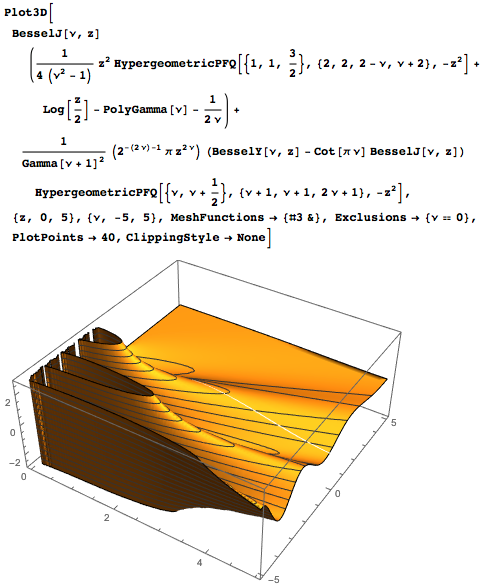For a fixed index, specifically v = 𝜋, we show the Bessel function J, together with its first two derivatives: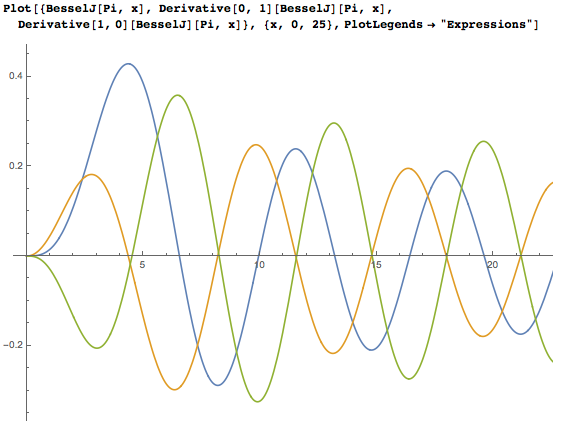It is interesting to note that the first two derivatives (with respect to z and with respect to v) have nearly the same zeros.

#### How did we get here?

It is remarkable that even almost 300 years after the introduction of a classical function (the Bessel function J0(z) was introduced by Daniel Bernoulli in 1732), it is still possible to find new and relatively simple formulas relating to such functions. The actual derivation of the formula introduced above for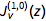(along with the related results for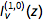, and the Neumann, Macdonald, and Kelvin functions) was complicated, and was achieved using the help of the Wolfram Language. Details of the derivation are published, and here we give a rough sketch of derivation of the approach that was used.

First, we recall that the Bessel and other functions in which we are interested for this program are of the hypergeometric type; differentiation by parameters of the generic hypergeometric function of a single variable pFq(a1, …, ap; b1, …, bq; z) requires more complicated functions of the hypergeometric type with more than one variable (see this article by L. U. Ancarani and G. Gasaneo). The first derivative with respect to an upper “parameter” ak, and all derivatives of symbolic integer order m with respect to a “lower” parameter bk of the generalized hypergeometric function, can be expressed in terms of the Kampé de Fériet hypergeometric function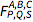of two variables by the following formulas: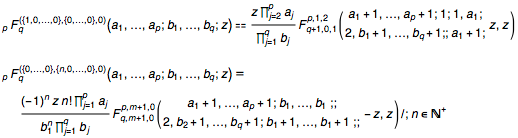Above, the Kampé de Fériet hypergeometric function is defined by the double series (see defining expressions here and here).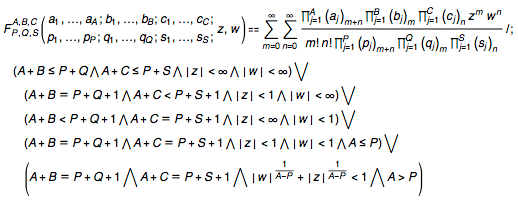The Kampé de Fériet function can be considered as a generalization of the hypergeometric function to two variables: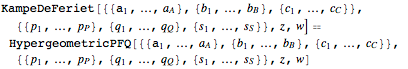A corresponding regularized version of the function can also be defined by replacing the product of Pochhammer symbols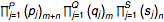in the denominator with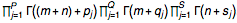The Kampé de Fériet function can be used to obtain a representation of the derivatives of the Bessel function J with respect to its parameter: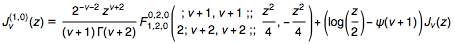This expression coincides with the simpler formula above, which involves hypergeometric functions of one variable, though this is not necessarily easy to see (we don’t yet have a fully algorithmic treatment for the reduction of multivariate hypergeometric functions into expressions containing only univariate hypergeometric functions, and this has contributed to the difficulty in discovering formulas like the one discussed here).

Double series, like the one given above defining the generalized hypergeometric functions of two variables, also arise in the evaluation of Mellin transforms of products of three Meijer G-functions: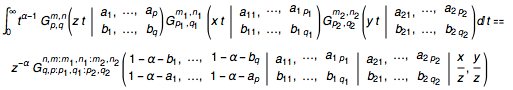The right side of this formula includes a Meijer G-function of two variables that generically can be represented, in the non-logarithmic case, as a finite sum of Kampé de Fériet hypergeometric functions with some coefficients, by analogy with these two formulas. Finally, the Kampé de Fériet function also arises in the separation of the real and imaginary parts of hypergeometric functions of one variable, z==x+ⅈy, with real arguments: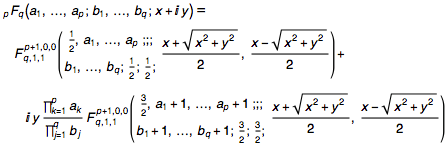It should be mentioned that in recent years the hypergeometric functions of many variables have found growing applications in the realms of quantum field theory, chemistry, engineering, and, in particular, communication theory and radio location. Many quite practical results can be represented using such functions, and consequently, most of the principal results in this field are obtained in the applied science literature. The theory of such functions in theoretical mathematics circles has thus far been elaborated relatively weakly.

#### Symbolic derivatives in the Wolfram Language

We are lucky here at Wolfram to have the originator of these new and exciting symbolic derivative formulas, Yury Brychkov, as part of our team, enabling us to bring this constantly developing area of research work into the grasp of our users. We are also lucky to have at our disposal the Entity framework of the Wolfram Language, which allows, among other things, for the integration of cutting-edge new results such as these on the timescale of weeks or days, in a computable format, easily accessible from a variety of Wolfram Language platforms. For example, in Mathematica, one can evaluate the following: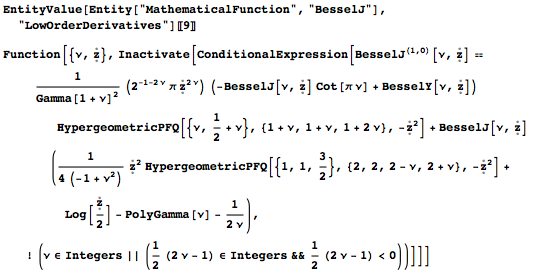This obtains the principal formula of this article. We can attempt to confirm the formula numerically by first substituting global values of v and z and activating; we get: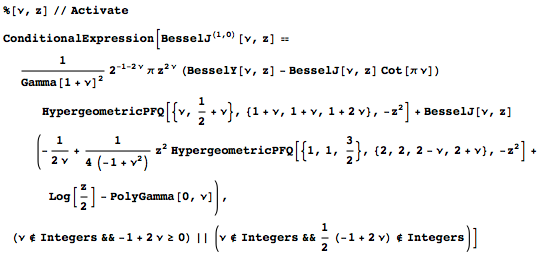Next, we separate the left- and right- hand sides (to allow for floating-point numerical errors) and substitute random values for the argument and parameter to obtain: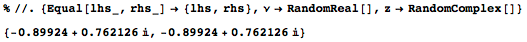The numerical derivative of the left-hand side is computed internally in the Wolfram Language via a limiting procedure. The equality of left- and right-hand sides, and therefore the correctness of the original derivative formula, is thus apparent.

Aside from the many new results for symbolic and parametric derivatives alluded to in this article and available only through EntityValue (though deeper integration into future versions of the Wolfram Language is an ongoing effort), a large number of long-standing results in this field have already been implemented into the Mathematica kernel and the core Wolfram Language. By reason of their complexity, such derivatives by parameters are not evaluated automatically, but can be seen using the FunctionExpand command. For example: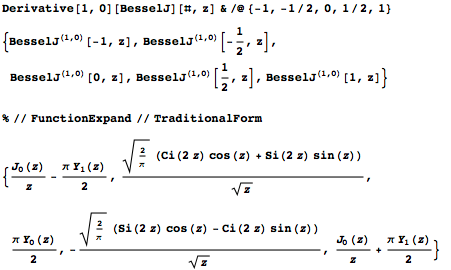At the second and higher order, derivatives of Bessel and related functions can still be expressed in terms of the Kampé de Fériet hypergeometric functionof two variables, but the resulting formulas can be rather complicated, and can include the Bell Y polynomials: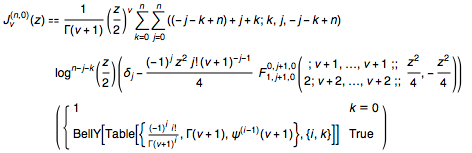The latter arise from expressing the Bessel function Jv(z) as the composition of the function
0F1(; v+1; w) and function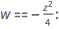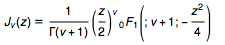We utilize Faà di Bruno’s formula, which describes the nth derivative of a composition of m functions fi(z)/; 1 ≤ im. In the m=2 case (see here and here), we obtain, for instance, an expression involving the following: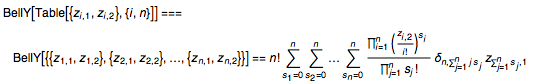The corresponding formula for generic m and n can be obtained and verified in the Wolfram Language as: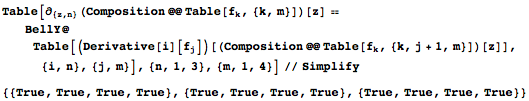While the Bell Y’s—for which no generic closed forms exist—are generally needed to express higher-order derivatives, as this blog was headed to press one of the authors, Yury Brychkov, even found a way to eliminate Bell Y from the nth derivatives with respect to the parameter of the Bessel functions, leaving us with the remarkable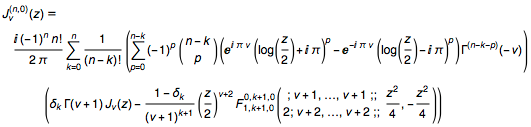For the convenience of interested users who would like to see in one place all known formulas for derivatives of special functions with respect to parameters (including those listed above), we have collected and presented these formulas in the following ways: